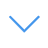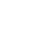# Solve Math

Solve Math is one of the Math Games that you can play on UGameZone.com for free. Are you sensitive to mathematical calculations? This game challenges the player's responsiveness and mathematical computing skills. You have to solve the equals answer by given random numbers and mathematical symbols.

Read Moreاضغط على 【Esc】 للخروج من وضع ملء الشاشة

# Solve Math

Solve Math is one of the Math Games that you can play on UGameZone.com for free. Are you sensitive to mathematical calculations? This game challenges the player's responsiveness and mathematical computing skills. You have to solve the equals answer by given random numbers and mathematical symbols.لتشغيل اللعبة ، تحتاج إلى السماح بتشغيل Adobe Flash Player في متصفحك.

You can use UGameZone Flash Enabler plugin to enable Flash automatically. Or you can follow our instructions to enable Flash manually.

play automatically

## Game Ratings

75%

يلعب 500

تقييم 75%

الأصوات 700

700
0
Category
Math Games
الكلمات
وصف

Solve Math is one of the Math Games that you can play on UGameZone.com for free. Are you sensitive to mathematical calculations? This game challenges the player's responsiveness and mathematical computing skills. You have to solve the equals answer by given random numbers and mathematical symbols.

Step 1
Step 2